# REASONING (Test 5)

Tag: reasoning
Q.1
What number should replace the question mark?A. 5
B. 8
C. 7
D. 1
Explaination / Solution:

The number formed at the top is half of the number formed at the bottom i.e. 358 x 2 = 716

Workspace
Report
Q.2
Four metal rods of lengths 78 cm, 104 cm, 117 cm and 169 cm are to be cut into parts of equal length. Each part must be as long as possible. What is the maximum number of pieces that can be cut?
A. 27
B. 36
C. 43
D. 480
Explaination / Solution:
No Explaination.

Workspace
Report
Q.3
Six persons A, B, C, D, E and F are standing in a row. C and D are standing close to each other alongside E. B is standing beside A only. A is fourth from F. Who are standing on the extremes?
A. A and F
B. B and D
C. B and F
D. None of the above
Explaination / Solution:
No Explaination.

Workspace
Report
Q.4
Two cars start towards each other, from two places A and B which are at a distance of 160 km. They start at the same time 08:10 AM. If the speeds of the cars are 50 km and 30 km per hour respectively, they will meet each other at
A. 10:10 AM
B. 10:30 AM
C. 11:10 AM
D. 11:20 AM
Explaination / Solution:
No Explaination.

Workspace
Report
Q.5
In a rare coin collection, there is one gold coin for every three non-gold coins. 10 more gold coins are added to the collection and the ratio of gold coins to non-gold coins would be 1: 2. Based on the information, the total number of coins in the collection now becomes?
A. 90
B. 80
C. 60
D. 50
Explaination / Solution:
No Explaination.

Workspace
Report
Q.6
Find the number of triangles in the given figure.A. 18
B. 20
C. 24
D. 27
Explaination / Solution:

The figure may be labelled as shown.The simplest triangles are IJO, BCJ, CDK, KQL, MLQ, GFM, GHN and NIO i.e. 8 in number.

The triangles composed of two components each are ABO, AHO, NIJ, IGP, ICP, DEQ, FEQ, KLM, LCP and LGP i.e.10 in number.

The triangles composed of four components each are HAB, DEF, LGI, GIC, ICL and GLC i.e. 6 in number.

Total number of triangles in the figure = 8 + 10 + 6 = 24.

Workspace
Report
Q.7
Find the number of triangles in the given figure.A. 10
B. 12
C. 14
D. 16
Explaination / Solution:

The figure may be labelled as shown.The simplest triangles are ABJ, ACJ, BDH, DHF, CIE and GIE i.e. 6 in number.

The triangles composed of two components each are ABC, BDF, CEG, BHJ, JHK, JKI and CJI i.e. 7 in number.

There is only one triangle JHI which is composed of four components.

Thus, there are 6 + 7 + 1 = 14 triangles in the given figure.

Workspace
Report
Q.8

What is the number of straight lines and the number of triangles in the given figure.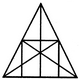A. 10 straight lines and 34 triangles
B. 9 straight lines and 34 triangles
C. 9 straight lines and 36 triangles
D. 10 straight lines and 36 triangles
Explaination / Solution:

The figure may be labelled as shown.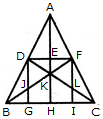The Horizontal lines are DF and BC i.e. 2 in number.

The Vertical lines are DG, AH and FI i.e. 3 in number.

The Slanting lines are AB, AC, BF and DC i.e. 4 in number.

Thus, there are 2 + 3 + 4 = 9 straight lines in the figure.

Now, we shall count the number of triangles in the figure.

The simplest triangles are ADE, AEF, DEK, EFK, DJK, FLK, DJB, FLC, BJG and LIC i.e. 10 in number.

The triangles composed of two components each are ADF, AFK, DFK, ADK, DKB, FCK, BKH, KHC, DGB and FIC i.e. 10 in number.

The triangles composed of three components each are DFJ and DFL i.e. 2 in number.

The triangles composed of four components each are ABK, ACK, BFI, CDG, DFB, DFC and BKC i.e. 7 in number.

The triangles composed of six components each are ABH, ACH, ABF, ACD, BFC and CDB i.e. 6 in number.

There is only one triangle i.e. ABC composed of twelve components.

There are 10 + 10 + 2 + 7 + 6+ 1 = 36 triangles in the figure.

Workspace
Report
Q.9

What is the minimum number of colours required to fill the spaces in the given diagram without any two adjacent spaces having the same colour?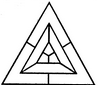A. 6
B. 5
C. 4
D. 3
Explaination / Solution:

The figure may be labelled as shown.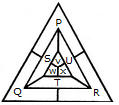The spaces P, Q and R have to be shaded by three different colours definitely (since each of these three spaces lies adjacent to the other two).

Now, in order that no two adjacent spaces be shaded by the same colour, the spaces T, U and S must be shaded with the colours of the spaces P, Q and R respectively.

Also the spaces X, V and W must be shaded with the colours of the spaces S, T and U respectively i.e. with the colours of the spaces R, P and Q respectively. Thus, minimum three colours are required.

Workspace
Report
Q.10

Count the number of squares in the given figure.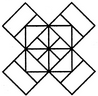A. 22
B. 20
C. 18
D. 14
Explaination / Solution:

The figure may be labelled as shown.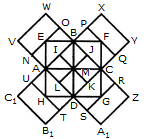The squares composed of two components each are BJMI, CKMJ, DLMK and AIML i.e. 4 in number.

The squares composed of three components each are EBMA, BFCM, MCGD and AMDH i.e. 4 in number.

The squares composed of four components each are VWBA, XYCB, ZA1DC and B1C1AD i.e. 4 in number.

The squares composed of seven components each are NOJL, PQKI, RSLJ and TUIK i.e. 4 in number.

There is only one square i.e. ABCD composed of eight components.

There is only one square i.e. EFGH composed of twelve components.

Total number of squares in the figure = 4 + 4 + 4 + 4 + 1 + 1 = 18.

Workspace
Report